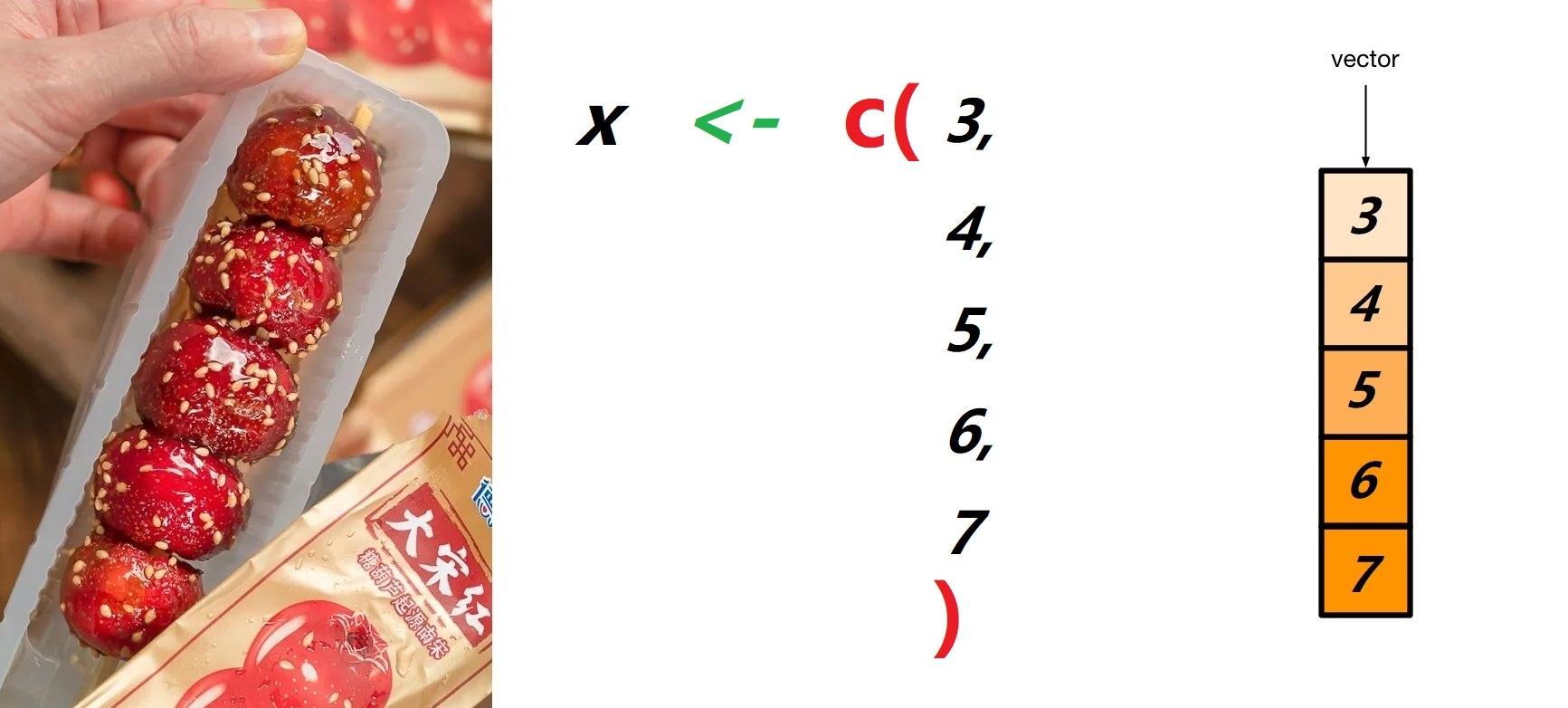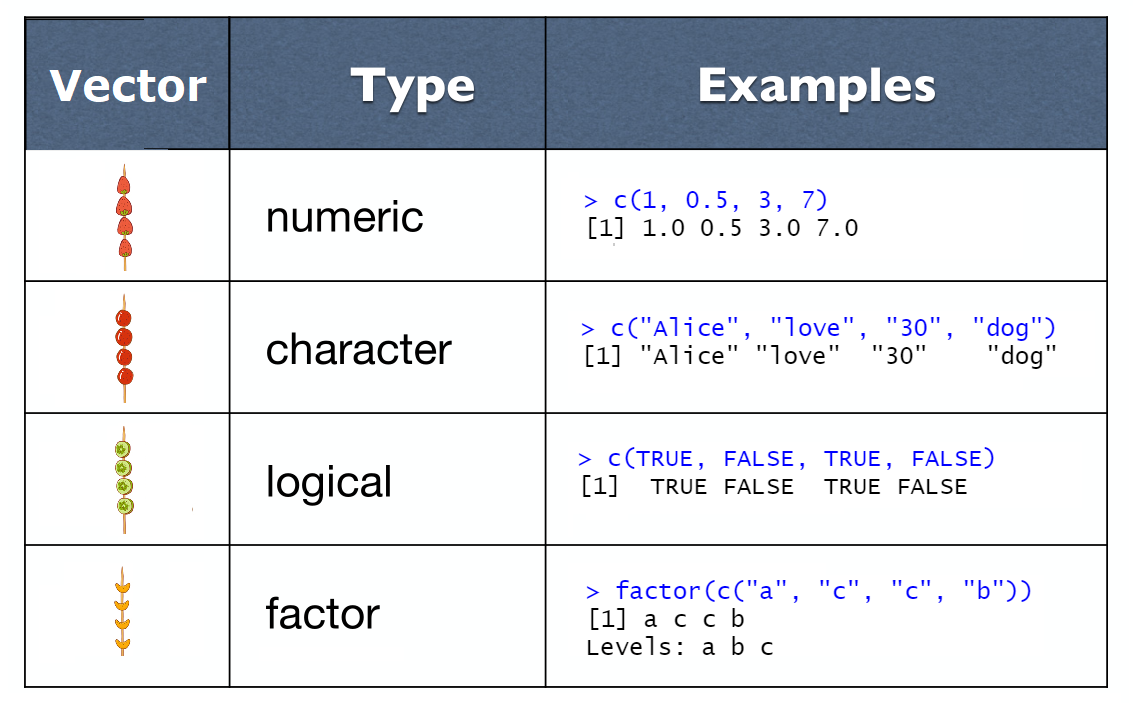# 第 4 章 向量

## 4.1 向量就像冰糖葫芦

x <- 6

x <- 3, 4, 5, 6, 7   # work？x <- c(3, 4, 5, 6, 7)
x
##  3 4 5 6 7

• 这里的c就是 combine 或 concatenate 的意思
• 它要求元素之间用英文的逗号分隔
• 且元素的数据类型是统一的，比如这里都是数值

### 4.1.1 聚合成新向量

c() 函数还可以把两个向量聚合成一个新的向量。

low      <- c(1, 2, 3)
high     <- c(4, 5, 6)
sequence <- c(low, high)
sequence
##  1 2 3 4 5 6

### 4.1.2 命名向量(named vector)

x <- c('a' = 5, 'b' = 6, 'c' = 7, 'd' = 8)
x
## a b c d
## 5 6 7 8

x <- c(5, 6, 7, 8)
names(x) <- c('a', 'b', 'c', 'd')
x
## a b c d
## 5 6 7 8

### 4.1.3 单个元素的向量

x <- 6

x <- c(6)

• 类型
x <- c(3, 4, 5, 6, 7)
typeof(x)
##  "double"
• 长度
length(x)
##  5

## 4.2 数值型向量

x <- c(1L, 5L, 2L, 3L)    # 整数型
x <- c(1.5, -0.5, 2, 3)   # 双精度类型，常用写法
x <- c(3e+06, 1.23e2)     # 双精度类型，科学计数法

• seq() 函数可以生成等差数列，from 参数指定数列的起始值，to 参数指定数列的终止值，by 参数指定数值的间距：
s1 <- seq(from = 0, to = 10, by = 0.5)
s1
##    0.0  0.5  1.0  1.5  2.0  2.5  3.0  3.5  4.0  4.5
##   5.0  5.5  6.0  6.5  7.0  7.5  8.0  8.5  9.0  9.5
##  10.0
• rep() 是 repeat（重复）的意思，可以用于产生重复出现的数字序列：x 用于重复的向量，times 参数可以指定要生成的个数，each 参数可以指定每个元素重复的次数
s2 <- rep(x = c(0, 1), times = 3)
s2
##  0 1 0 1 0 1
s3 <- rep(x = c(0, 1), each = 3)
s3
##  0 0 0 1 1 1
• m:n，如果单纯是要生成数值间距为1的数列，用 m:n 更快捷，它产生从 m 到 n 的间距为1的数列
s4 <- 0:10  # Colon operator (with by = 1):
s4
##    0  1  2  3  4  5  6  7  8  9 10
s5 <- 10:1
s5
##   10  9  8  7  6  5  4  3  2  1

## 4.3 字符串型向量

x <- c("a", "b", "c")
x <- c('Alice', 'Bob', 'Charlie', 'Dave')
x <- c("hello", "baby", "I love you!") 

x1 <- c("1", "2", "3")
x2 <- c(1, 2, 3)

## 4.4 逻辑型向量

x <- c(TRUE, TRUE, FALSE, FALSE)
x <- c(T, T, F, F)            # Equivalent, but not recommended

TRUE和FALSE必须都大写，不能写成下面这些形式

x <- c(True, False)
x <- c(true, false)          

x1 <- c(TRUE, FALSE)             # logical
x2 <- c("TRUE", "FALSE")         # character

## 4.5 因子型向量

four_seasons <- c("spring", "summer", "autumn", "winter")
four_seasons
##  "spring" "summer" "autumn" "winter"

four_seasons_factor <- factor(four_seasons)
four_seasons_factor
##  spring summer autumn winter
## Levels: autumn spring summer winter

four_seasons <- c("spring", "summer", "autumn", "winter")
four_seasons_factor <- factor(four_seasons,
levels = c("summer", "winter", "spring", "autumn")
)
four_seasons_factor
##  spring summer autumn winter
## Levels: summer winter spring autumn

soldier <- factor(c("Colonel", "General", "Captain"),
levels = c("General", "Colonel", "Captain")
)
soldier
##  Colonel General Captain
## Levels: General Colonel Captain

## 4.6 小结## 4.7 强制转换

• character > numeric > logical
• double > integer

c(1, "foo", TRUE)
##  "1"    "foo"  "TRUE"

c(7, TRUE, FALSE)
##  7 1 0

c(1L, 2, pi)
##  1.000 2.000 3.142

## 4.8 习题

• 请说出fun3的结果
fun <- c("programming", "in", "R")
fun2 <- c("Have", "fun")
fun3 <- c(fun2, fun)
fun3
• 数据类型必须一致是构建向量的基本要求，如果数值型、字符串型和逻辑型写在一起，用c()函数构成向量，猜猜会发生什么？
x <- c(1, "USA", TRUE)
x
• 形容温度的文字
temperatures <- c("warm", "hot", "cold")